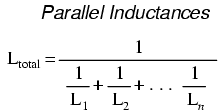Home   >   Textbooks   >   Lessons In Electric Circuits   >   Vol. I - DC   >   Inductors   >   Series and Parallel Inductors

Chapter 15: INDUCTORS

# Series and Parallel Inductors

When inductors are connected in series, the total inductance is the sum of the individual inductors' inductances. To understand why this is so, consider the following: the definitive measure of inductance is the amount of voltage dropped across an inductor for a given rate of current change through it. If inductors are connected together in series (thus sharing the same current, and seeing the same rate of change in current), then the total voltage dropped as the result of a change in current will be additive with each inductor, creating a greater total voltage than either of the individual inductors alone. Greater voltage for the same rate of change in current means greater inductance.Thus, the total inductance for series inductors is more than any one of the individual inductors' inductances. The formula for calculating the series total inductance is the same form as for calculating series resistances:When inductors are connected in parallel, the total inductance is less than any one of the parallel inductors' inductances. Again, remember that the definitive measure of inductance is the amount of voltage dropped across an inductor for a given rate of current change through it. Since the current through each parallel inductor will be a fraction of the total current, and the voltage across each parallel inductor will be equal, a change in total current will result in less voltage dropped across the parallel array than for any one of the inductors considered separately. In other words, there will be less voltage dropped across parallel inductors for a given rate of change in current than for any of those inductors considered separately, because total current divides among parallel branches. Less voltage for the same rate of change in current means less inductance.Thus, the total inductance is less than any one of the individual inductors' inductances. The formula for calculating the parallel total inductance is the same form as for calculating parallel resistances:• REVIEW: